## Saturday, 9 August 2014

### CHAPTER 2 - Binomial Theorem for Positive Integral Index

Let us now consider more formally the binomial theorem. We need to expand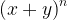${\left( {x + y} \right)^n}$, where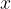$x$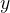$y$ are two arbitrary quantities, but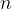$n$ is a positive integer.
In particular, if we write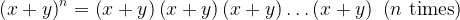${\left( {x + y} \right)^n} = \left( {x + y} \right)\left( {x + y} \right)\left( {x + y} \right)\ldots \left( {x + y} \right) \ \left( {n\,\,{\rm{times}}} \right)$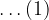$\ldots(1)$
we need to find out the coefficient of${x^i}{y^j}$. Note that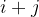$i + j$ must always equal$n$, so that we can write a general term of the expansion (without the coefficient) as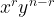${x^r}{y^{n - r}}$ so that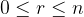$0 \le r \le n$.
Now, to find the coefficient of${x^r}{y^{n - r}}$, note that we need the quantity$x$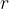$r$times, while$y$ is needed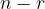$n - r$ times. Thus, in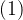$(1)$${x^r}{y^{n - r}}$ will be formed whenever$x$ is ‘contributed’ by$r$ of the binomial terms, while$y$ is ‘contributed’ by the remaining$n - r$ of the binomial terms. For example, in the expansion of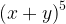${\left( {x + y} \right)^5}$, to form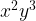${x^2}{y^3}$, we need$x$ from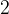$2$ terms and$y$ from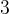$3$:How many ways are there to form${x^2}{y^3}$? In other words, how many times will${x^2}{y^3}$ be formed? The number of times${x^2}{y^3}$ is formed is what is the coefficient of${x^2}{y^3}$. That number, which would be immediately obvious to the alert reader, is simply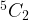$^5{C_2}$. Why? Because this is the number of ways in which we can select any$2$ binomial terms from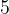$5$. These$2$ terms will contribute$x$. The remaining will automatically contribute$y$.
In the general case of${\left( {x + y} \right)^n}$, we see that the coefficient of${x^r}{y^{n - r}}$ would be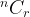$^n{C_r}$. (which is infact the same as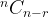$^n{C_{n - r}}$). Thus, the general binomial expansion isThe coefficients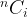$^n{C_i}$ are called the binomial coefficients, for a reason that should now be obvious.
Note that the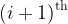${\left( {i + 1} \right)^{{\rm{th}}}}$ coefficient in this expansion is$^n{C_i}$, which now explains the relation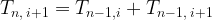${T_{n,\,i + 1}} = {T_{n - 1,i}} + {T_{n - 1,\,i + 1}}$
we observed in the Pascal triangle; this relation simply corresponds to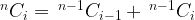$^n{C_i} = {\,^{n - 1}}{C_{i - 1}} + {\,^{n - 1}}{C_i}$
Also, the binomial coefficients of terms equidistant from the beginning and the end are equal, because we have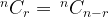$^n{C_r} = {\,^n}{C_{n - r}}$. The general term of expansion,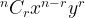$^n{C_r}{x^{n - r}}{y^r}$, is the${(r + 1)^{th}}$ term from the beginning of the expansion and is conventionally denoted by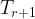${T_{r + 1}}$, i.e.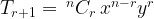${T_{r + 1}} = {\,^n}{C_r}\,{x^{n - r}}{y^r}$
Since we have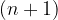$(n + 1)$ terms in the general expansion, we see that if$n$ is even, there will be an odd number of terms, and thus there will be only one middle term, which would be$^n{C_{n/2}}\,{x^{n/2}}{y^{n/2}}$. For example,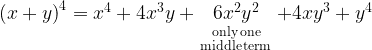${\left( {x + y} \right)^4} = {x^4} + 4{x^3}y + \mathop {6{x^2}{y^2}}\limits_{\scriptstyle\,\,\,\,\,{\rm{only}}\,{\rm{one}}\hfill\atop \scriptstyle{\rm{middle}}\,{\rm{term}}\hfill} + 4x{y^3} + {y^4}$
On the other hand, if$n$ is odd , then there will be an even number of terms in the expansion, and thus there will be two middle terms, namely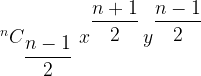$^n{C_{\dfrac{{n - 1}}{2}}}\,\,{x^{\dfrac{{n + 1}}{2}}}\,{y^{\dfrac{{n - 1}}{2}}}$ and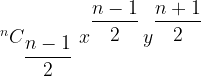$^n{C_{\dfrac{{n - 1}}{2}}}\,\,{x^{\dfrac{{n - 1}}{2}}}\,{y^{\dfrac{{n + 1}}{2}}}$
For example;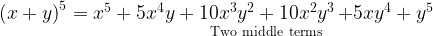${\left( {x + y} \right)^5} = {x^5} + 5{x^4}y + \mathop {10{x^3}{y^2} + 10{x^2}{y^3}}\limits_{{\rm{Two}}\,\,{\rm{middle}}\,\,\,{\rm{terms}}} + 5x{y^4} + {y^5}$